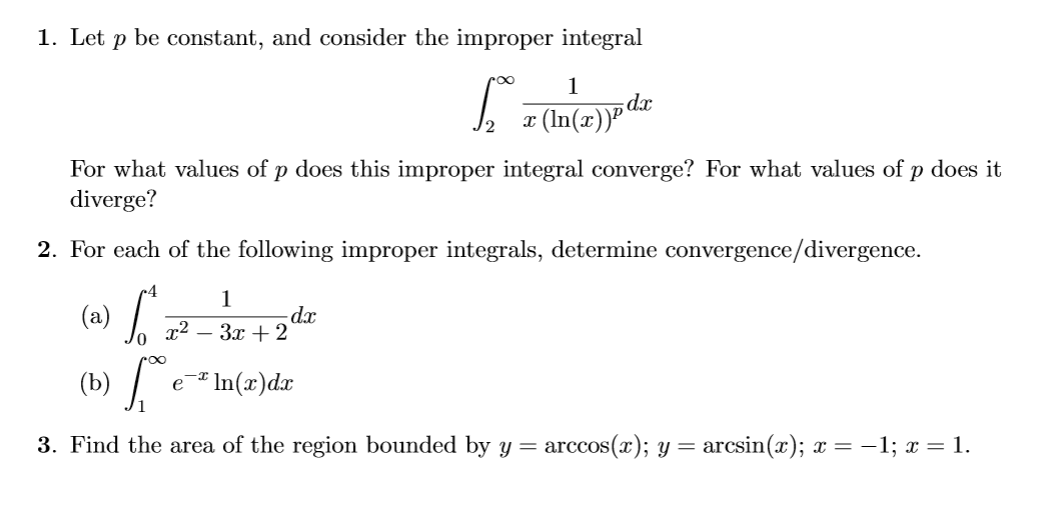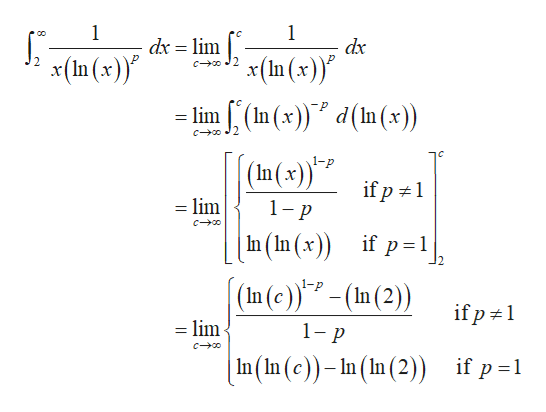# 1. Let p be constant, and consider the improper integral1(In(r)PFor what values of p does this improper integral converge? For what values of p does itdiverge?2. For each of the following improper integrals, determine convergence/divergence.1- da(a)In(x)dac(b)3. Find the area of the region bounded by y = arccos(x); y = arcsin(x); x = -1;1

Question
32 views

Lost with improper integrals. Need help. Thank you!help_outlineImage Transcriptionclose1. Let p be constant, and consider the improper integral 1 (In(r)P For what values of p does this improper integral converge? For what values of p does it diverge? 2. For each of the following improper integrals, determine convergence/divergence. 1 - da (a) In(x)dac (b) 3. Find the area of the region bounded by y = arccos(x); y = arcsin(x); x = -1; 1 fullscreen
check_circle

star
star
star
star
star
1 Rating
Step 1

Hey, since there are multiple questions posted, we will answer first question. If you want any specific question to be answered then please submit that question only or specify the question number in your message

Step 2

Evaluate the given improper ...help_outlineImage Transcriptionclose1 dx lim 1 dx x(n(x) x(n(x) =lim(In (x)) d(In (x)) co 2 co2 (In(x) 1-p if p 1 lim 1-p Co In (In (x)) if p 1 (In (c)- (In (2) lim if p 1 1- p C0 In(In (c))-n (In (2)) if p 1 fullscreen

### Want to see the full answer?

See Solution

#### Want to see this answer and more?

Solutions are written by subject experts who are available 24/7. Questions are typically answered within 1 hour.*

See Solution
*Response times may vary by subject and question.
Tagged in

### Math